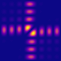QPMS Electromagnetic multiple scattering library and toolkit.
Overview of QPMS command line utilities

The utilities are located in the misc directory. Run the utility with -h argument to get more info.

# Rectangular and square 2D lattices

These scripts deal with simple 2D rectangular lattices, finite or infinite, one scatterer per unit cell. $$D_{2h}$$ or $$D_{4h}$$ symmetric adapted bases are used where applicable.

## Finite lattices

• finiterectlat-modes.py: Search for resonances using Beyn's algorithm.
• finiterectlat-scatter.py: Plane wave scattering.
• finiterectlat-constant-driving.py: Rectangular array response to a driving where a subset of particles are excited by basis VSWFs with the same phase.

## Infinite lattices

• rectlat_simple_modes.py: Search for lattice modes using Beyn's algorithm.
• infiniterectlat-k0realfreqsvd.py: Evaluate the lattice mode problem singular values at the Γ point for a real frequency interval. Useful as a starting point in lattice mode search before using Beyn's algorithm.
• infiniterectlat-scatter.py: Plane wave scattering.

# General 2D lattices

## Infinite lattices

These can contain several scatterers per unit cell. Symmetry adapted bases currently not implemented.

• lat2d_modes.py: Search for lattice modes using Beyn's algorithm.
• lat2d_realfreqsvd.py: Evaluate the lattice mode problem singular values at the Γ point for a real frequency interval. Useful as a starting point in lattice mode search before using Beyn's algorithm.